# 直推和主动机器学习中的梯度提升

10 三月 2021, 08:29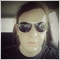0
548

### 介绍

"在解决感兴趣的问题时，不要把解决更一般的问题作为中间步骤。试着得到你真正需要的答案，而不是更笼统的答案。"

"我们将得出这样一个结论：苏格拉底是凡人，如果我们把我们的论证纯粹归纳起来，而不是用“人人都是凡人”的方式，然后用演绎的方式，那么苏格拉底就更接近于确定性".

• 连续性假设。彼此接近的点更有可能共享一个标签。在有监督学习中也假设了这一点，并且产生了一种偏好，即分离类的几何简单边界。在半监督学习的情况下，平滑性假设在低密度区域中产生偏好，在低密度区域中，很少有点彼此接近，但在不同的类中。
• 集群假设。数据往往会形成离散的簇，同一簇中的点更有可能共享一个标签（尽管共享一个标签的数据可以分布在多个簇中）。这是光滑性假设的一个特例，导致使用聚类算法进行学习。
• 流形假设。数据大致位于比输入空间低得多的维数的流形上。在这种情况下，使用标记和未标记的数据学习流形可以避免维数灾难。然后利用流形上定义的距离和密度继续学习。

• 一些邻近度量（例如，欧几里德距离）用于基于标记的数据区域（伪标签）标记其余的数据,
• 训练标签与伪标签和符号相结合。
• 模型在整个数据集上进行训练。

• 成员查询综合. 在这种情况下，模型从某个分布中生成一个实例，该分布对所有示例都是公共的。这可能是一个带有附加噪声的类实例，也可能只是所讨论空间中的一个似是而非的点。这个新点被送到先知（oracle)那里培训，Oracle 是估值函数的传统名称，用于评估模型的给定功能实例的值。
• 基于流的采样. 根据这个场景，每次检查一个未标记的数据点，然后 Oracle 根据某种信息标准选择是要查询这个点的类标签还是拒绝它。
• 基于池的采样. 在这个场景中，有大量未标记的示例，就像前面的例子一样,实例是根据信息量从池中选择的,从池中选择信息量最大的实例。这是活跃的学习爱好者中最流行的情景。将对所有未标记的实例进行排序，然后选择信息量最大的实例。

• 不确定度抽样（最小置信度）。根据这个策略，我们选择模型最不确定的实例。例如，将标签分配给某个类的概率低于某个边界。
• 边际抽样. 第一种策略的缺点是它只决定属于一个标签的概率，而忽略属于其他标签的概率。边际抽样策略选择两个最可能的标签之间的最小概率差。
• 熵采样. 对每个实例应用熵公式，查询出最大值的实例。

• 该模型是在标记数据上训练的。
• 同样的模型用于标记未标记的数据以预测概率（伪标记）。
• 选择新实例查询策略。
• 根据信息量从数据池中选取N个实例加入训练样本。
• 重复此循环，直到达到某个停止标准。停止标准可以是迭代次数或学习误差的估计，也可以是其他外部准则。

### 主动学习

Python语言中有几个用于主动学习的库，其中最流行的是：

• modAL 是一个非常简单易学的包，它是流行的机器学习库 sciket learn 的一种包装（它们完全兼容）。该软件包提供了最流行的主动学习方法。
• Libact使用多臂老虎机(multi-armed bandit)策略来动态选择最佳查询。
• Alipy 是一种来自包提供商的实验室，包含了大量的查询策略。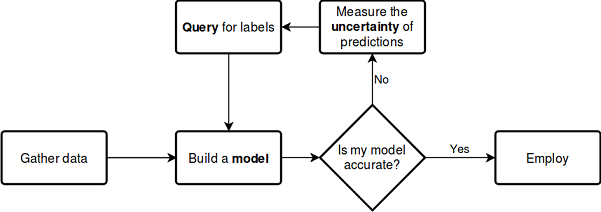```from modAL.models import ActiveLearner
from modAL.uncertainty import entropy_sampling
from sklearn.ensemble import RandomForestClassifier

learner = ActiveLearner(
estimator=RandomForestClassifier(),
query_strategy=entropy_sampling,
X_training=X_training, y_training=y_training
)
```

modAL 库支持查询策略的简单组合，并允许从中生成复合加权策略：

```from modAL.utils.combination import make_linear_combination, make_product
from modAL.uncertainty import classifier_uncertainty, classifier_margin

# creating new utility measures by linear combination and product
# linear_combination will return 1.0*classifier_uncertainty + 1.0*classifier_margin
linear_combination = make_linear_combination(
classifier_uncertainty, classifier_margin,
weights=[1.0, 1.0]
)
# product will return (classifier_uncertainty**0.5)*(classifier_margin**0.1)
product = make_product(
classifier_uncertainty, classifier_margin,
exponents=[0.5, 0.1]
)
```

```from modAL.utils.selection import multi_argmax

# defining the custom query strategy, which uses the linear combination of
# classifier uncertainty and classifier margin
def custom_query_strategy(classifier, X, n_instances=1):
utility = linear_combination(classifier, X)
query_idx = multi_argmax(utility, n_instances=n_instances)
return query_idx, X[query_idx]

custom_query_learner = ActiveLearner(
estimator=GaussianProcessClassifier(1.0 * RBF(1.0)),
query_strategy=custom_query_strategy,
X_training=X_training, y_training=y_training
)
```

### 查询策略

```[[0.1 , 0.85, 0.05],
[0.6 , 0.3 , 0.1 ],
[0.39, 0.61, 0.0 ]]

1 - proba.max(axis=1)

[0.15, 0.4 , 0.39]
```

```>>> import numpy as np
>>> proba = np.array([[0.1 , 0.85, 0.05],
...                   [0.6 , 0.3 , 0.1 ],
...                   [0.39, 0.61, 0.0 ]])
>>>
>>> proba
array([[0.1 , 0.85, 0.05],
[0.6 , 0.3 , 0.1 ],
[0.39, 0.61, 0.  ]])
>>> part = np.partition(-proba, 1, axis=1)
>>> part
array([[-0.85, -0.1 , -0.05],
[-0.6 , -0.3 , -0.1 ],
[-0.61, -0.39, -0.  ]])
>>> part[:, 0]
array([-0.85, -0.6 , -0.61])
>>> part[:, 1]
array([-0.1 , -0.3 , -0.39])
>>> margin = - part[:, 0] + part[:, 1]
>>> margin
array([0.75, 0.3 , 0.22])
```

`[0.51818621, 0.89794572, 0.66874809]`

### 主动学习

```def active_learner(data, labeled_size, unlabeled_size, batch_size, max_depth):
X_raw = data[data.columns[1:-1]].to_numpy()
y_raw = data[data.columns[-1]].to_numpy()

# Isolate our examples for our labeled dataset.
training_indices = np.random.randint(low=0, high=X_raw.shape + 1, size=labeled_size)

X_train = X_raw[training_indices]
y_train = y_raw[training_indices]

# fit the model on all data
cl = AdaBoostClassifier(DecisionTreeClassifier(max_depth=max_depth), n_estimators=50, learning_rate = 0.01)
cl.fit(X_raw, y_raw)
print('Score for the passive learning: ', cl.score(X_raw, y_raw), ' with train size: ', data.shape)

# Isolate the non-training examples we'll be querying.
X_pool = np.delete(X_raw, training_indices, axis=0)
y_pool = np.delete(y_raw, training_indices, axis=0)

# Pre-set our batch sampling to retrieve 3 samples at a time.
preset_batch = partial(uncertainty_batch_sampling, n_instances=batch_size)

# Specify our core estimator along with its active learning model.
cl = AdaBoostClassifier(DecisionTreeClassifier(max_depth=3), n_estimators=50, learning_rate = 0.03)
learner = ActiveLearner(estimator=cl, query_strategy=preset_batch, X_training=X_train, y_training=y_train)
```

```    # Allow our model to query our unlabeled dataset for the most
# informative points according to our query strategy (uncertainty sampling).
N_QUERIES = unlabeled_size // batch_size

for index in range(N_QUERIES):
query_index, query_instance = learner.query(X_pool)

# Teach our ActiveLearner model the record it has requested.
X, y = X_pool[query_index], y_pool[query_index]
learner.teach(X=X, y=y)

# Remove the queried instance from the unlabeled pool.
X_pool, y_pool = np.delete(
X_pool, query_index, axis=0), np.delete(y_pool, query_index)

# Calculate and report our model's accuracy.
model_accuracy = learner.score(X_raw, y_raw)
print('Accuracy after query {n}: {acc:0.4f}'.format(
n=index + 1, acc=model_accuracy))

# Save our model's performance for plotting.
performance_history.append(model_accuracy)

print('Score for the active learning with train size: ',
learner.X_training.shape)
```

```>>> learned = active_learner(pr, 1000, 1000, 50)
Score for the passive learning:  0.5991245668429692  with train size:  5483
Accuracy after query 1: 0.5710
Accuracy after query 2: 0.5836
Accuracy after query 3: 0.5749
Accuracy after query 4: 0.5847
Accuracy after query 5: 0.5829
Accuracy after query 6: 0.5823
Accuracy after query 7: 0.5650
Accuracy after query 8: 0.5667
Accuracy after query 9: 0.5854
Accuracy after query 10: 0.5836
Accuracy after query 11: 0.5807
Accuracy after query 12: 0.5907
Accuracy after query 13: 0.5944
Accuracy after query 14: 0.5865
Accuracy after query 15: 0.5949
Accuracy after query 16: 0.5873
Accuracy after query 17: 0.5833
Accuracy after query 18: 0.5862
Accuracy after query 19: 0.5902
Accuracy after query 20: 0.6002
Score for the active learning with train size:  (2000, 8)
```

```# prepare data for CatBoost
catboost_df = pd.DataFrame(learned.X_training)
catboost_df['labels'] = learned.y_training

# perform GMM clusterization over dataset
X = catboost_df.copy()
gmm = mixture.GaussianMixture(
n_components=75, max_iter=500, covariance_type='full', n_init=1).fit(X)

# sample new dataset
generated = gmm.sample(10000)
# make labels
gen = pd.DataFrame(generated)
gen.rename(columns={gen.columns[-1]: "labels"}, inplace=True)
gen.loc[gen['labels'] >= 0.5, 'labels'] = 1
gen.loc[gen['labels'] < 0.5, 'labels'] = 0
X = gen[gen.columns[:-1]]
y = gen[gen.columns[-1]]
pr = pd.DataFrame(X)
pr['labels'] = y

# fit CatBoost model and test it
model = fit_model(pr)
test_model(model, TEST_START, END_DATE)
```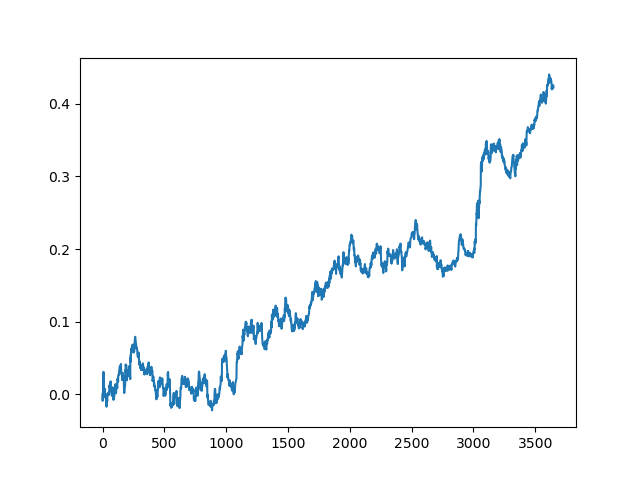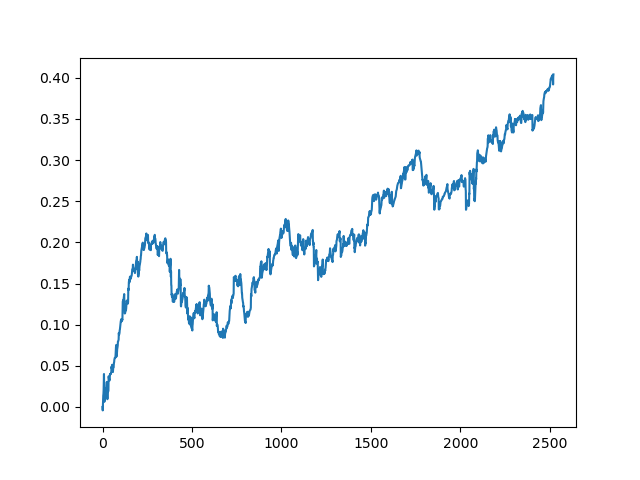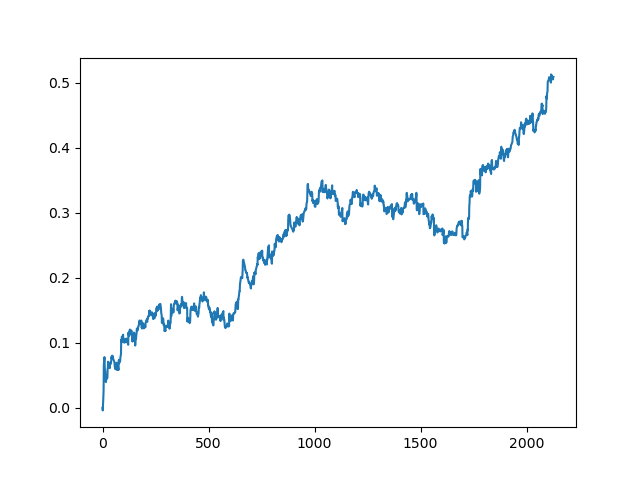```def active_learner_committee(data, learners_number, labeled_size, unlabeled_size, batch_size):
X_pool = data[data.columns[1:-1]].to_numpy()
y_pool = data[data.columns[-1]].to_numpy()

cl = AdaBoostClassifier(DecisionTreeClassifier(max_depth=3), n_estimators=50, learning_rate = 0.05)
cl.fit(X_pool, y_pool)
print('Score for the passive learning: ', cl.score(
X_pool, y_pool), ' with train size: ', data.shape)

# initializing Committee members
learner_list = list()

# Pre-set our batch sampling to retrieve 3 samples at a time.
preset_batch = partial(uncertainty_batch_sampling, n_instances=batch_size)

for member_idx in range(learners_number):
# initial training data
train_idx = np.random.choice(range(X_pool.shape), size=labeled_size, replace=False)
X_train = X_pool[train_idx]
y_train = y_pool[train_idx]

# creating a reduced copy of the data with the known instances removed
X_pool = np.delete(X_pool, train_idx, axis=0)
y_pool = np.delete(y_pool, train_idx)

# initializing learner
learner = ActiveLearner(
estimator=AdaBoostClassifier(DecisionTreeClassifier(max_depth=2), n_estimators=50, learning_rate = 0.05),
query_strategy=preset_batch,
X_training=X_train, y_training=y_train
)
learner_list.append(learner)

# assembling the committee
committee = Committee(learner_list=learner_list)

unqueried_score = committee.score(X_pool, y_pool)
performance_history = [unqueried_score]

N_QUERIES = unlabeled_size // batch_size

for idx in range(N_QUERIES):
query_idx, query_instance = committee.query(X_pool)
committee.teach(
X=X_pool[query_idx].reshape(1, -1),
y=y_pool[query_idx].reshape(1, )
)
model_accuracy = committee.score(X_pool, y_pool)
performance_history.append(model_accuracy)
print('Accuracy after query {n}: {acc:0.4f}'.format(
n=idx + 1, acc=model_accuracy))

# remove queried instance from pool
X_pool = np.delete(X_pool, query_idx, axis=0)
y_pool = np.delete(y_pool, query_idx)

return committee
```

```>>> committee = active_learner_committee(pr, 5, 1000, 1000, 50)
Score for the passive learning:  0.6533842794759825  with train size:  5496
Accuracy after query 1: 0.5927
Accuracy after query 2: 0.5818
Accuracy after query 3: 0.5668
Accuracy after query 4: 0.5862
Accuracy after query 5: 0.5874
Accuracy after query 6: 0.5906
Accuracy after query 7: 0.5918
Accuracy after query 8: 0.5910
Accuracy after query 9: 0.5820
Accuracy after query 10: 0.5934
Accuracy after query 11: 0.5864
Accuracy after query 12: 0.5753
Accuracy after query 13: 0.5868
Accuracy after query 14: 0.5921
Accuracy after query 15: 0.5809
Accuracy after query 16: 0.5842
Accuracy after query 17: 0.5833
Accuracy after query 18: 0.5783
Accuracy after query 19: 0.5732
Accuracy after query 20: 0.5828
```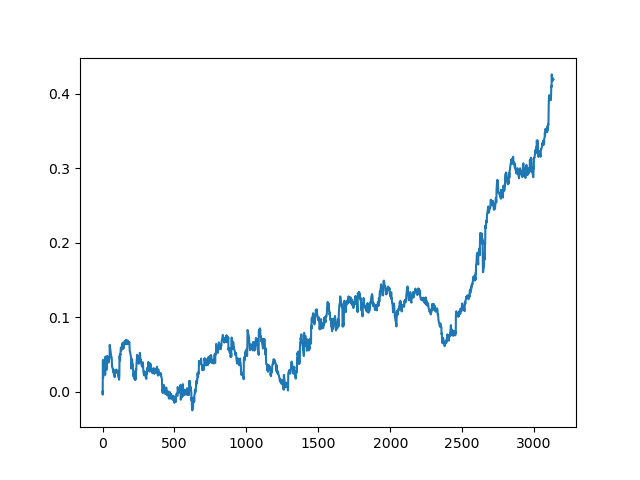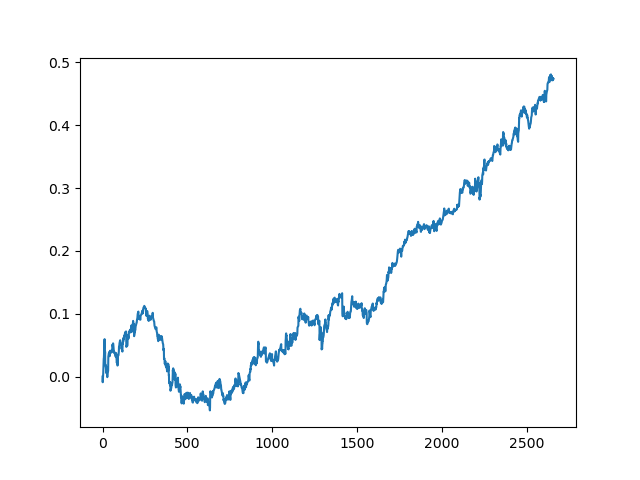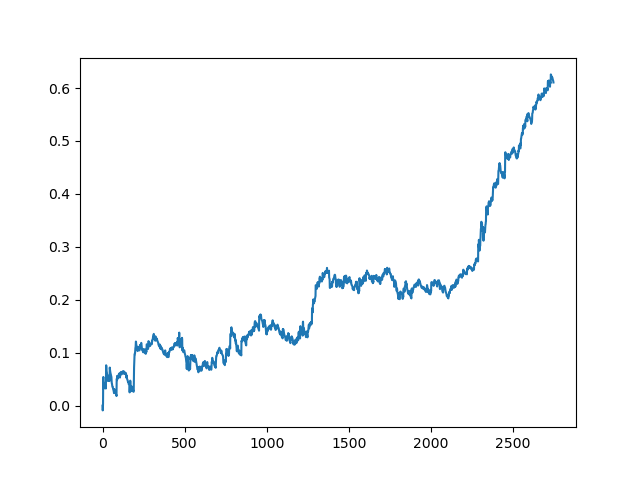### 结论

#### 该作者的其他文章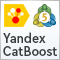利用 CatBoost 算法寻找外汇市场的季节性模式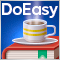DoEasy 函数库中的时间序列（第五十七·部分）：存储一次即时报价数据的对象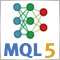神经网络变得轻松（第九部分）：操作归档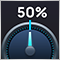开发自适应算法 (第二部分): 提高效率